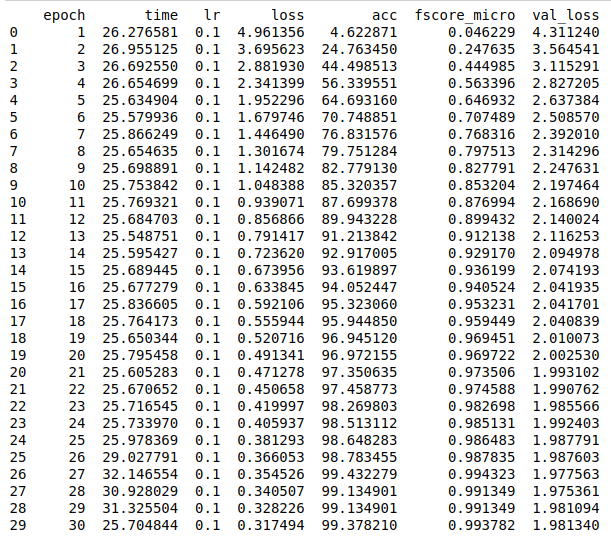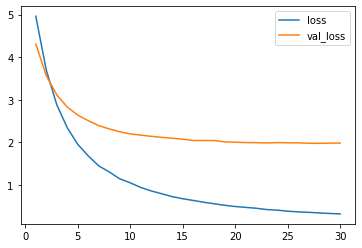# Transfer learning example

Note

But first, let’s import all the needed packages.

```import os
import tarfile
import urllib.request

import matplotlib.pyplot as plt
import numpy as np
import pandas as pd
from sklearn.model_selection import train_test_split
import torch
import torch.nn as nn
import torch.optim as optim
import torchvision.models as models
from torch.utils import model_zoo
from torchvision import transforms
from torchvision.datasets import ImageFolder

from poutyne import set_seeds, Model, ModelCheckpoint, CSVLogger, Experiment
```

Also, we need to set Pythons’s, NumPy’s and PyTorch’s seeds by using Poutyne function so that our training is (almost) reproducible.

```set_seeds(42)
```

```def download_and_extract_dataset(path):
os.makedirs(path, exist_ok=True)
tgz_filename = os.path.join(path, "images.tgz")

urllib.request.urlretrieve("https://graal.ift.ulaval.ca/public/CUB200.tgz", tgz_filename)
print("Extracting archive...")
archive = tarfile.open(tgz_filename)
archive.extractall(path)
```
```base_path = './datasets/CUB200'
extract_dest = os.path.join(base_path, 'images')
```

We create our dataset object.

```norm_coefs = {}
norm_coefs['cub200'] = [(0.47421962,  0.4914721 ,  0.42382449), (0.22846779,  0.22387765,  0.26495799)]
norm_coefs['imagenet'] = [(0.485, 0.456, 0.406), (0.229, 0.224, 0.225)]
transform = transforms.Compose([
transforms.Resize((224,224)),
transforms.ToTensor(),
transforms.Normalize(*norm_coefs['cub200'])
])

# is_valid_file removes hidden files from the dataset.
dataset = ImageFolder(extract_dest, transform=transform,
is_valid_file=lambda path: not os.path.split(path).startswith('.'))
```

We do the split train/valid/test with a 60/20/20 split respectively. We do a stratified split with scikit-learn in order to get examples of every class in every split.

```# We take 60% of the dataset for the training dataset
train_indices, valid_test_indices = train_test_split(np.arange(len(dataset)),
train_size=0.6,
stratify=dataset.targets,
random_state=42)
# We take 20% for the validation dataset and 20% for the test dataset
# (i.e. 50% of the remaining 40%).
valid_indices, test_indices = train_test_split(valid_test_indices,
train_size=0.5,
stratify=np.asarray(dataset.targets)[valid_test_indices],
random_state=42)

train_dataset = Subset(dataset, train_indices)
valid_dataset = Subset(dataset, valid_indices)
test_dataset = Subset(dataset, test_indices)
```

Now, let’s set our training constants. We first have the CUDA device used for training if one is present. Second, we set the number of classes (i.e. one for each number). Finally, we set the batch size (i.e. the number of elements to see before updating the model), the learning rate for the optimizer, and the number of epochs (i.e. the number of times we see the full dataset).

```cuda_device = 0
device = torch.device("cuda:%d" % cuda_device if torch.cuda.is_available() else "cpu")

num_classes = 200
batch_size = 32
learning_rate = 0.1
n_epoch = 30
```

Creation of the PyTorch’s dataloader to split our data into batches.

```train_loader = DataLoader(train_dataset, batch_size=batch_size, num_workers=8, shuffle=True)
```

We load a pretrained `ResNet-18` networks and replace the head with the number of neurons equal to our number of classes.

```resnet18 = models.resnet18(pretrained=True)
resnet18.fc = nn.Linear(resnet18.fc.in_features, num_classes)
```

We freeze the network except for its head.

```def freeze_weights(resnet18):
for name, param in resnet18.named_parameters():
if not name.startswith('fc.'):

freeze_weights(resnet18)
```

We define callbacks for saving last epoch, best epoch and logging the results.

```# We are saving everything into ./saves/cub200.
save_base_dir = 'saves'
save_path = os.path.join(save_base_dir, 'cub200')
os.makedirs(save_path, exist_ok=True)

callbacks = [
# Save the latest weights to be able to resume the optimization at the end for more epochs.
ModelCheckpoint(os.path.join(save_path, 'last_epoch.ckpt')),

# Save the weights in a new file when the current model is better than all previous models.
ModelCheckpoint(os.path.join(save_path, 'best_epoch_{epoch}.ckpt'), monitor='val_acc', mode='max',
save_best_only=True, restore_best=True, verbose=True),

# Save the losses and accuracies for each epoch in a TSV.
CSVLogger(os.path.join(save_path, 'log.tsv'), separator='\t'),
]
```

Finally, we start the training and output its final test loss, accuracy, and micro F1-score.

Note

The F1-score is quite similar to the accuracy since the dataset is very balanced.

```optimizer = optim.SGD(resnet18.fc.parameters(), lr=learning_rate, weight_decay=0.001)
loss_function = nn.CrossEntropyLoss()

model = Model(resnet18, optimizer, loss_function,
batch_metrics=['accuracy'], epoch_metrics=['f1'],
device=device)

print('Test:\n\tLoss: {}\n\tAccuracy: {}\n\tF1-score: {}'.format(test_loss, test_acc, test_f1))
```
```logs = pd.read_csv(os.path.join(save_path, 'log.tsv'), sep='\t')
print(logs)

best_epoch_idx = logs['val_acc'].idxmax()
best_epoch = int(logs.loc[best_epoch_idx]['epoch'])
print("Best epoch: %d" % best_epoch)
``````metrics = ['loss', 'val_loss']
plt.plot(logs['epoch'], logs[metrics])
plt.legend(metrics)
plt.show()
``````metrics = ['acc', 'val_acc']
plt.plot(logs['epoch'], logs[metrics])
plt.legend(metrics)
plt.show()
```Since we have created checkpoints using callbacks, we can restore the best model from those checkpoints and test it.

```resnet18 = models.resnet18(pretrained=False, num_classes=num_classes)

model = Model(resnet18, 'sgd', 'cross_entropy',
batch_metrics=['accuracy'], epoch_metrics=['f1'],
device=device)

print('Test:\n\tLoss: {}\n\tAccuracy: {}\n\tF1-score: {}'.format(test_loss, test_acc, test_f1))
```

We can also use the `Experiment` class to train our network. This class saves checkpoints and logs as above in a directory and allows to stop and resume optimization at will. See `documentation` for details.

```def experiment_train(epochs):
# Reload the pretrained network and freeze it except for its head.
resnet18 = models.resnet18(pretrained=True)
resnet18.fc = nn.Linear(resnet18.fc.in_features, num_classes)
freeze_weights(resnet18)

# Saves everything into ./saves/cub200_resnet18_experiment
save_path = os.path.join(save_base_dir, 'cub200_resnet18_experiment')

optimizer = optim.SGD(resnet18.fc.parameters(), lr=learning_rate, weight_decay=0.001)

# Poutyne Experiment
exp = Experiment(save_path, resnet18, device=device, optimizer=optimizer, task='classif')

# Train

# Test
```experiment_train(epochs=5)
```experiment_train(epochs=10)